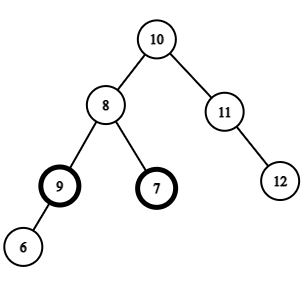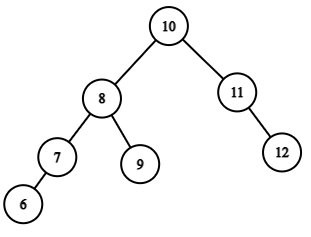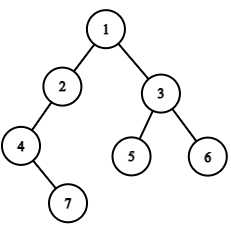# Ninja And The Tree

Posted: 23 Mar, 2021
Difficulty: Hard

## PROBLEM STATEMENT

#### A binary search tree (BST) is a binary tree data structure with the following properties.

``````• The left subtree of a node contains only nodes with data less than the node’s data.
• The right subtree of a node contains only nodes with data greater than the node’s data.
• Both the left and right subtrees must also be binary search trees.
``````
##### Example``````This is the binary search tree made by Ninja. But here, the left node of 8 is greater, and the right node is smaller.
If we swap the right and the left node of 8 Ninja’s Binary Search Tree will become correct.
````````````This is the correct binary Search Tree.
``````
##### Input Format
``````The first line contains an Integer 'T' which denotes the number of test cases or queries to be run. Then the test cases follow.

The first line of each test case contains the elements of the tree in the level order form separated by a single space.

If any node does not have a left or right child, take -1 in its place. Refer to the example below.
``````
##### Example
``````Elements are in the level order form. The input consists of values of nodes separated by a single space in a single line. In case a node is null, we take -1 in its place.
For example, the input for the tree depicted in the below image would be :
````````````1
2 3
4 -1 5 6
-1 7 -1 -1 -1 -1
-1 -1

Explanation :
Level 1 :
The root node of the tree is 1

Level 2 :
Left child of 1 = 2
Right child of 1 = 3

Level 3 :
Left child of 2 = 4
Right child of 2 = null (-1)
Left child of 3 = 5
Right child of 3 = 6

Level 4 :
Left child of 4 = null (-1)
Right child of 4 = 7
Left child of 5 = null (-1)
Right child of 5 = null (-1)
Left child of 6 = null (-1)
Right child of 6 = null (-1)

Level 5 :
Left child of 7 = null (-1)
Right child of 7 = null (-1)

The first not-null node (of the previous level) is treated as the parent of the first two nodes of the current level. The second not-null node (of the previous level) is treated as the parent node for the next two nodes of the current level and so on.

The input ends when all nodes at the last level are null (-1).
``````
##### Note:
``````The above format was just to provide clarity on how the input is formed for a given tree.

The sequence will be put together in a single line separated by a single space. Hence, for the above-depicted tree, the input will be given as:

1 2 3 4 -1 5 6 -1 7 -1 -1 -1 -1 -1 -1
``````
##### Output Format:
``````For each test case, print the recovered correct Binary Search Tree in level order form.

Print the output of each test case in a separate line.
``````
##### Note:
``````You do not need to print anything, it has already been taken care of. Just implement the given function.
``````
##### Constraints:
``````1 <= T <= 100
2 <= N <= 5000
0 <= X <= 2^31 - 1

Where 'N' is the number of nodes in the BST and 'X' is the value at the node.

Time limit: 1 sec
``````Approach 1

Algorithm:

1. We will initialize an array of integers to store the inorder traversal of the given BST.
2. As two nodes are swapped currently, so the inorder traversal will not be sorted.
3. Sort the array.
4. Now, this is a sorted array so this will represent the inorder traversal of the BST.
5. Insert the array elements back to the tree inorder manner.